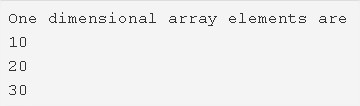# One Dimensional Array In Java – Tutorial & Example

One Dimensional Array Program in Java – In this article, we will detail in on all the different methods to describe the one-dimensional array program in Java with suitable examples & sample outputs.

• Using Standard Method
• Using Scanner
• Using String

An array is a collection of elements of one specific type in a horizontal fashion. The array in contention here is that of the one-dimensional array in Java programming.

Anything having one-dimension means that there is only one parameter to deal with. In regular terms, it is the length of something. Similarly, as far as an array is concerned, one dimension means it has only one value per location or index.

One-dimensional array in Java programming is an array with a bunch of values having been declared with a single index.As you can see in the example given above, firstly, you need to declare the elements that you want to be in the specified array.

Secondly, the location of each element needs to particularized as well, since that is where the elements will be stored respectively.

• Thirdly, you need to declare the array accordingly.

As it is visible, the three elements that have been listed simultaneously are as follows:

• 10
• 20
• 30

Hence, the result is printed as 10 20 30 respectively in single lines, since it is a one-dimensional array.

Thus, the methods used to do so in Java are as follows:

## One Dimensional Array – Using Standard Method

Output:

### Using Scanner

1. Read the array length as sc.nextInt() and store it in the variable len and declare an array int[len].

2) To store elements in to the array for i=0 to i<length of an array read the element using sc.nextInt() and store the element at the index a[i].

3) Display the elements of an array for loop iterates from i=0 to i<length of an array print the array element a[i].

Output:

#### Using For Loop – One Dimensional Array

Output:

##### Using String
1. We declared one-dimensional string array with the elements strings.

2) To print strings from the string array. for i=0 to i<length of the string print string which is at the index str[i].

Output:

x

## Java Program to Add Two Matrices – 4 Ways | Programs

Java program to add two matrices – The following Java Code will let you know ...